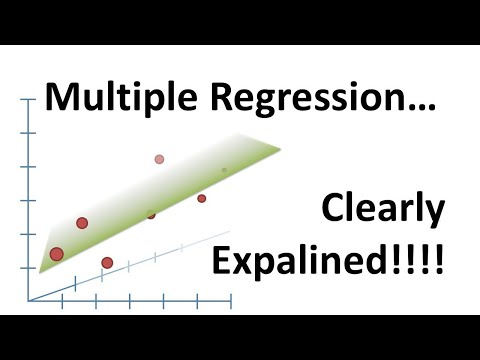Promowane transkrypcje

TRANSKRYPCJA VIDEO# Multiple Regression, Clearly Explained!!!

### Opis wygenerowany przez Skrybot

Dla tego filmu nie została wybrana usługa automatycznego generowania opisu. Wciąż można to zrobić wybierając odpowiednie opcje sekcji Premium.

### Transkrypcja wygenerowana przez Skrybot

StackQuest, StackQuest, StackQuest, StackQuest, yeah, StackQuest. Hello! I'm Josh Stommer and welcome to StackQuest. StackQuest is brought to you by the friendly folks in the genetics department at the University of North Carolina at Chapel Hill. Today we're going to be talking about multiple regression, and it's going to be clearly explained! This StackQuest builds on the one for linear regression, so if you haven't already seen that one yet, check it out. Alright, now let's get to it. People who don't understand linear regression tend to make a big deal out of the differences between simple and multiple regression. It's not a big deal, and the StackQuest on simple linear regression already covered most of the concepts we're going to cover here.

You might recall from the StackQuest on linear regression that simple regression is just fitting a line to data. We're interested in the R squared and the P value to evaluate how well that line fits the data. In that same StackQuest, I also showed you how to fit a plane to data. Well, that's what multiple regression is. You fit a plane or some higher dimensional object to your data. A term like higher dimensional object sounds really fancy and complicated, but it's not. All it means is that we're adding additional data to the model.

In the previous example, all that meant was that instead of just modeling body length by mouse weight, we modeled body length using mouse weight and tail length. If we added additional factors, like the amount of food eaten or the amount of time spent running on a wheel, well, those would be considered additional dimensions, but they're really just additional pieces of data that we can add to our fancy equation. So from the StackQuest on linear regression, you may remember the first thing we did was calculate R squared. Well, the good news is calculating R squared is the exact same for both simple regression and multiple regression. There's absolutely no difference.

Here's the equation for R squared, and we plug in the values for the sums of squares around the fit, and then we plug in the sums of squares around the mean value for the body length. Regardless of how much additional data we add to our fancy equation, if we're using it to predict body length, then we use the sums of squares around the body length. One caveat is for multiple regression, you adjust R squared to compensate for the additional parameters in the equation. We covered this in the StackQuest for linear regression, so it's no big deal. Now we want to calculate a P value for our R squared. Calculating F and the P value is pretty much the same.

You plug in the sums of squares around the fit, and then you plug in the sums of squares around the mean. For simple regression, P fit equals 2, because we have two parameters in the equation that least squares has to estimate. And for this specific example, the multiple regression version of P fit equals 3, because least squares had to estimate three different parameters. If we added additional data to the model, for example, the amount of time a mouse spends running on a wheel, then we have to change P fit to equal the number of parameters in our new equation.

And for both simple regression and multiple regression, P mean equals 1, because we only have to estimate the mean value of the body length. So far, we have compared this simple regression to the mean, and this multiple regression to the mean. But we can compare them to each other, and this is where multiple regression really starts to shine. This will tell us if it's worth the time and trouble to collect the tail length data, because we will compare a fit without it, the simple regression, to a fit with it, the multiple regression. Calculating the F value is the exact same as before, only this time we replace the mean stuff with the simple regression stuff.

So instead of plugging in the sums of squares around the mean, we plug in the sums of squares around the simple regression. And instead of plugging in P mean, we plug in P simple, which equals the number of parameters in the simple regression. That's 2. And then we plug in the sums of squares for the multiple regression, and we plug in the number of parameters in our multiple regression equation. Bam! If the difference in R squared values between the simple and multiple regression is big, and the P value is small, then adjusting tail length to the model is worth the trouble. Hooray! We've made it to the end of another exciting stack quest.

Now for this stack quest, I've made another one that shows you how to do multiple regression in R. It shows all the little details and sort of what's important and what's not important about the output that R gives you. So check that one out, and don't forget to subscribe. OK, until next time, quest on!.

##### S K R Y B O T

Transkrypcje

###### Skrybot. 2023, Transkrypcje video z YouTube

Informujemy, że odwiedzając lub korzystając z naszego serwisu, wyrażasz zgodę aby nasz serwis lub serwisy naszych partnerów używały plików cookies do przechowywania informacji w celu dostarczenie lepszych, szybszych i bezpieczniejszych usług oraz w celach marketingowych.